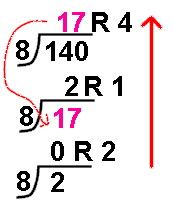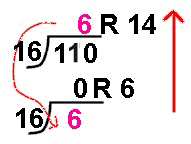Change a number from base 10
to any other base:

 Let's see if we can convert some numbers from one base to another.  There are many ways to work with number base conversions.  We will be discussing only one method.  Please feel free to use any conversion system with which you are comfortable. Don't panic!  Yes, it is math.  But you can do it!Example 1:  Convert 510 (read 5 base 10) into base 2.The Process:
1.  Divide the "desired" base (in this case base 2) INTO the number you are trying to convert.
2.  Write the quotient (the answer) with a remainder like you did in elementary school.
3.  Repeat this division process using the whole number from the previous quotient (the number in front of the remainder).
4.  Continue repeating this division until the number in front of the remainder is only zero.

510 = 1012  (a binary conversion)

Example 2:  Convert 14010 to base 8.The process is the same as in example 1.  The answer is:

14010 = 2148
(an octal conversion)

Example 3:  Convert 11010 to base 16.The process remains the same.  BUT there is one problem in base 16 that did not appear in the examples above.  One of the remainders in this division contains 2 digits (14).  You CANNOT allow 2 digits to reside in one of the place holdings in a number.  For this reason, base 16, which can have six 2-digit remainders (10, 11, 12, 13, 14, 15) replaces these values with alphabetic representations (10-A, 11-B, 12-C, 13-D, 14-E, 15-F).  The answer is:

11010 = 6E16